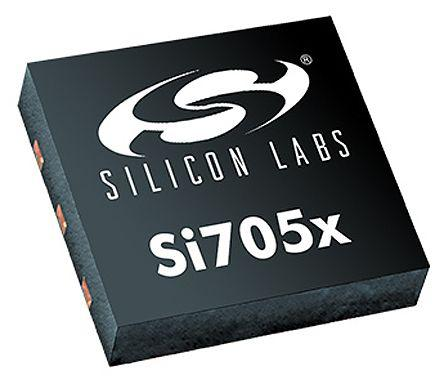# Current-Sensing Techniques for DC-DC Converters

DC-DC converters are electronic circuits used to change the DC power from one voltage level to another. Sometimes we need to increase or reduce the DC voltage to meet the requirements of various gadgets or components within a circuit. For instance, DC-DC converters are required to convert 24V from a truck’s battery to 12 to run a car stereo and to convert the 5V DC on PC motherboards need to be converted to 3V or less required to run some of the recent CPU chips.

DC is not as easy to step down as AC which only needs a transformer. DC-DC converters require current sensors to detect and convert current to the desired voltage which is proportional to the current through a measured path. There are different types of sensors, each with different performance, current range and environmental requirements. Listed below are different sensing techniques for DC-DC converters and their principles of operation.

## Resistor current sensing

It is the most common way of sensing current. This technique involves inserting a sense resistor in series with an inductor. This can be either surface mount or through hole resistors. The value of the resistor is used to determine the current through the inductor by sensing voltage across it. This method of sensing has been illustrated in the figure below,Some of the commonly used current sensing resistors include WSBS8518L1000JK, WSBS8515L100JK, MSC1632-3264, FCSL 64-90, LVK 12-25 and WLA-WLC. There are also shunt resistor ICs that are specifically designed for detecting overcurrent, for instance, the INA300, AD 8211, and AD8214.

• This technique exhibits very high accuracy

• A lot of power is dissipated

### Sensing

This technique incorporates the use of MOSFETS. They behave like resistors when they are “on” and biased in the non-saturated region. If small drain-source voltage is applied as it the case for MOSFETS when they are utilized as switches, there results in resistance equivalent to;

R_DS =  L/(WuC_ox (V_(GS-V_T)

Where u,  and  represent mobility, oxide capacitance per unit area and threshold voltage respectively.  After establishing  you use it to find the switch current by sensing voltage across the MOSFETS drain-source.The MOSFET’s  value is relatively insensitive to drain current, whereas it increases with increase in junction temperature. To compensate for this, MOSFET drivers like the AUIR3200S are used. Some of the commonly used MOSFETS for current sensing include TDA21320, TDA21321, Si6862DQ, Si4730EY, SUM50N03-13L and SUM60N08-07C among others.

• This technique is lossless hence it is power efficient.

• The technique has low accuracy since  is inherently nonlinear. Moreover, the  for on-chip MOSFETs exhibit substantial variation due to  and .  also varies substantially with temperature resulting in variance of between 50% and 100%.

### Filter-Sense the Inductor

A low-pass RC network is used to filter the voltage across an inductor and the current is sensed through an equivalent series resistance (ESR) of the inductor. Below is an illustration of how this worksVoltage across the inductor is equivalent to,

V_l=(R_l+sL)

The values of L and  have to be known so that R and C can be chosen accordingly.

• It is lossless.

• This technique is inappropriate for IC’s due the tolerance of the components needed to effect it.

### Observer Approach

An inductor voltage is used to measure current using their relationship as shown by the equation below;

V=Ldi/dt

Inductor current can then have established by passing the voltage through an Integrator over a period of time. For this technique to be efficient, the value of L ought to be known.• It is lossless

• The value of L ought to be known

### Current transformers

This technique is mostly used in high power systems. The essence is to sense a portion of the high inductor current using the mutual induction characteristic of the transformer.

• It is lossless

• It’s costly
• It requires a lot of space owing to its size
• Transformers cannot transfer the DC portion of the current

### SENSEFETs

A current FET is built in parallel with a MOSFET and its ability to measure used in sensing current as illustrated below;The W of the MOSFET used to sense is substantially smaller than that of the power FET. The W of the power MOSFET should be 100 and more times that of the SENSEFET. This guarantee that the power consumed in the SENSEFET is low. Both the voltages of M and S should be equal, so as to avoid mirror non-ideals due to channel- length modulation. Such effects can be mitigated with the help of current conveyors. The complete current sensing a SENSEFET is illustrated in the figure below,

The accuracy of the circuit decreases with an increase in the width ratio of both the main MOSFET and SENSEFET.

Some of the commonly used SENSEFETS include BUK7105-40AIE, BUK7C10-75AITE, and IXTN660N04T4 among others.

• It is lossless
• It offers moderate accuracy
• It’s very practical

• It has very low bandwidth
• Matching W presents a lot of challenges
• Requires the use of special MOSFETs

The kind of current sensing technique to be used in any application is selected based DC-DC converter control scheme. For instance, if voltage-mode control is to be used current sensing would only be needed for overcurrent protection hence simple, fairly accurate and lossless method  sensing would be ideal.

Resistor current sensing can be used where power loss is not critical for instance, in PCs where a power efficiency of approximately 5% is not critical. It is, therefore, to first understand the requirements of your application before picking the appropriate current sensing technique.